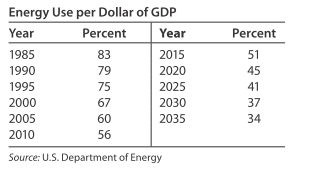# Energy use Energy use per dollar of GDP in the United States indexed to 1980 means that energy use for any year is viewed as a percent of the use per dollar of GDP in 1980. The table shows the energy use per dollar of GDP, as a percent, for selected years from 1985 and projected to 2035. Suppose the rate of change of energy use per dollar of GDP can be modeled by percentage points per year, where t is the number of years past 1980. d E d t = 0.00468 ( 0.4 t + 2 ) 2 − 0.104 ( 0.4 t + 2 ) − 0.56 percentage points per year, where t is the number of years past 1980. Use integration and the data point for 1990 to find the function E ( t ) that models the energy use per dollar of GDP. Use two significant digits. Let t = 0 represent 1980 and graph the model from part (a) with the data in the table. Find the model’s predicted energy use per dollar of GDP in 2025.### Mathematical Applications for the ...

11th Edition
Ronald J. Harshbarger + 1 other
Publisher: Cengage Learning
ISBN: 9781305108042

#### Solutions

Chapter
Section### Mathematical Applications for the ...

11th Edition
Ronald J. Harshbarger + 1 other
Publisher: Cengage Learning
ISBN: 9781305108042
Chapter 12.2, Problem 58E
Textbook Problem
1 views

## Energy use Energy use per dollar of GDP in the United States indexed to 1980 means that energy use for any year is viewed as a percent of the use per dollar of GDP in 1980. The table shows the energy use per dollar of GDP, as a percent, for selected years from 1985 and projected to 2035. Suppose the rate of change of energy use per dollar of GDP can be modeled by percentage points per year, where t is the number of years past 1980. d E d t = 0.00468 ( 0.4 t + 2 ) 2 − 0.104 ( 0.4 t + 2 ) − 0.56 percentage points per year, where t is the number of years past 1980.Use integration and the data point for 1990 to find the function E ( t ) that models the energy use per dollar of GDP. Use two significant digits. Let t = 0 represent 1980 and graph the model from part (a) with the data in the table. Find the model’s predicted energy use per dollar of GDP in 2025.

This textbook solution is under construction.

### Still sussing out bartleby?

Check out a sample textbook solution.

See a sample solution

#### The Solution to Your Study Problems

Bartleby provides explanations to thousands of textbook problems written by our experts, many with advanced degrees!

Get Started